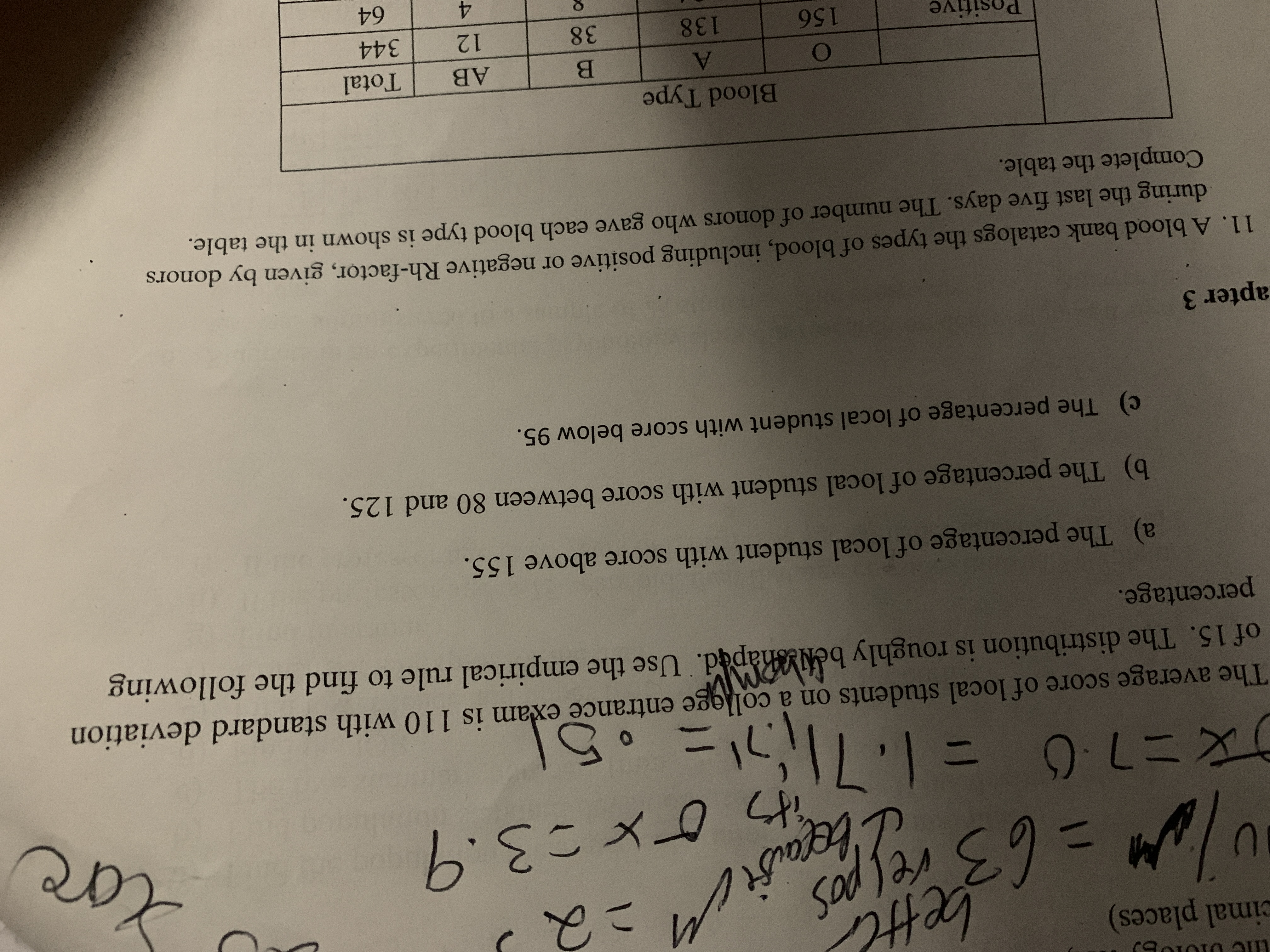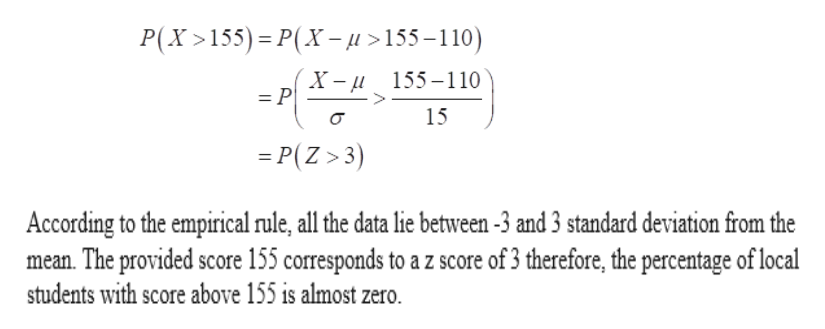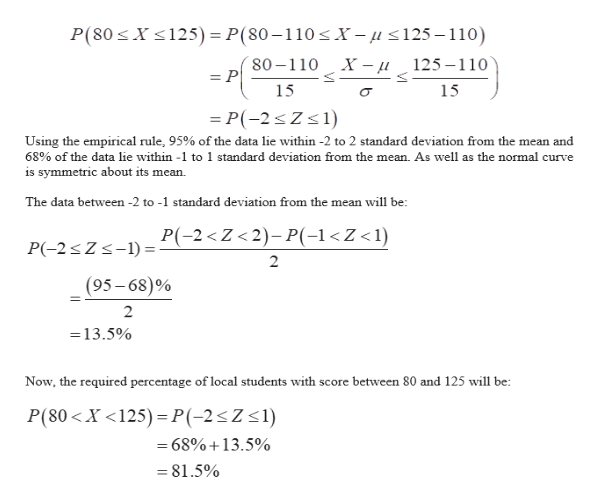# r9o1010 Aucimal places)sadleCx03.963The average score of local students on a college entrance exam is 110 with standard deviationof 15. The distribution is roughly belhaped. Use the empirical rule to find the followingpercentage.a) The percentage of local student with score above 155.b) The percentage of local student with score between 80 and 125.c) The percentage of local student with score below 95apter 311. A blood bank catalogs the types of blood, including positive or negative Rh-factor, given by donorsduring the last five days. The number of donors who gave each blood type is shown in the table.Complete the table.Blood TypeTotalАВЯ3834412138156Positive64

Questionhelp_outlineImage Transcriptioncloser9o1010 Au cimal places) sadle Cx03.9 63 The average score of local students on a college entrance exam is 110 with standard deviation of 15. The distribution is roughly belhaped. Use the empirical rule to find the following percentage. a) The percentage of local student with score above 155. b) The percentage of local student with score between 80 and 125. c) The percentage of local student with score below 95 apter 3 11. A blood bank catalogs the types of blood, including positive or negative Rh-factor, given by donors during the last five days. The number of donors who gave each blood type is shown in the table. Complete the table. Blood Type Total АВ Я 38 344 12 138 156 Positive 64 fullscreen
check_circle

Step 1

From the provided information, the average (μ) and standard deviation (σ) of local students on a college entrance exam are 110 and 15 respectively.

Consider X be a random variable which represents the score of local students on a college entrance exam.

a).  Now, the required percentage of local students with score above 155 can be calculated as:help_outlineImage TranscriptioncloseP(X>155) P(X-u> 155 -1 10) X- и 155-110 = P 15 =P(Z>3) According to the empirical rule, all the data lie between -3 and 3 standard deviation from the mean. The provided score 155 corresponds to a z score of 3 therefore, the percentage of local students with score above 155 is almost zero. b fullscreen
Step 2

b). The percentage of local students with score ...help_outlineImage TranscriptioncloseX 125) = P(80 -110 X- u <125-110) P(80 80-110 X - u 125-110 = P 15 15 P(-2 Z s 1) Using the empirical rule, 95% of the data lie within -2 to 2 standard deviation from the mean and 68% of the data lie within -1 to 1 standard deviation from the mean. As well as the normal curve is symmetric about its mean The data between -2 to -1 standard deviation from the mean will be P(-2 < Z < 2)-P(-1

### Want to see the full answer?

See Solution

#### Want to see this answer and more?

Solutions are written by subject experts who are available 24/7. Questions are typically answered within 1 hour.*

See Solution
*Response times may vary by subject and question.
Tagged in

### Statistics